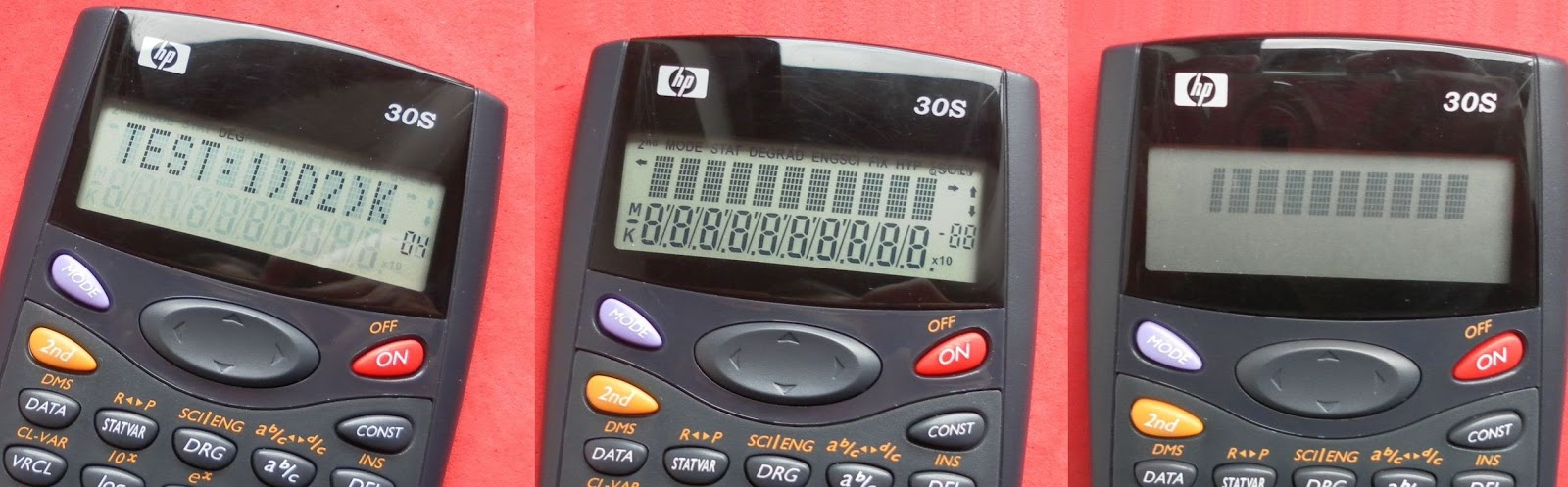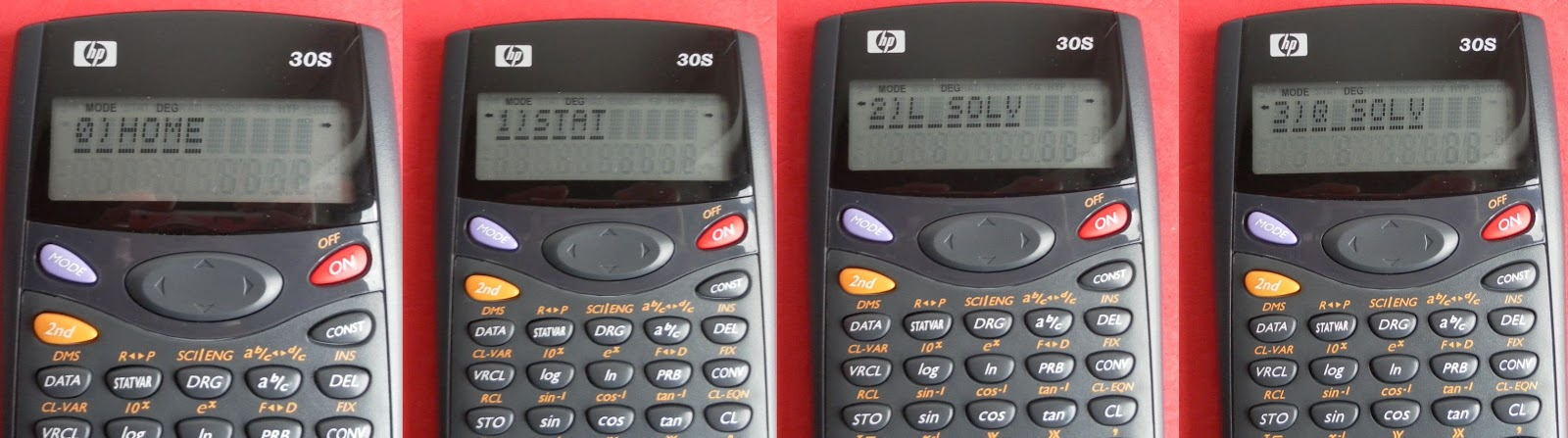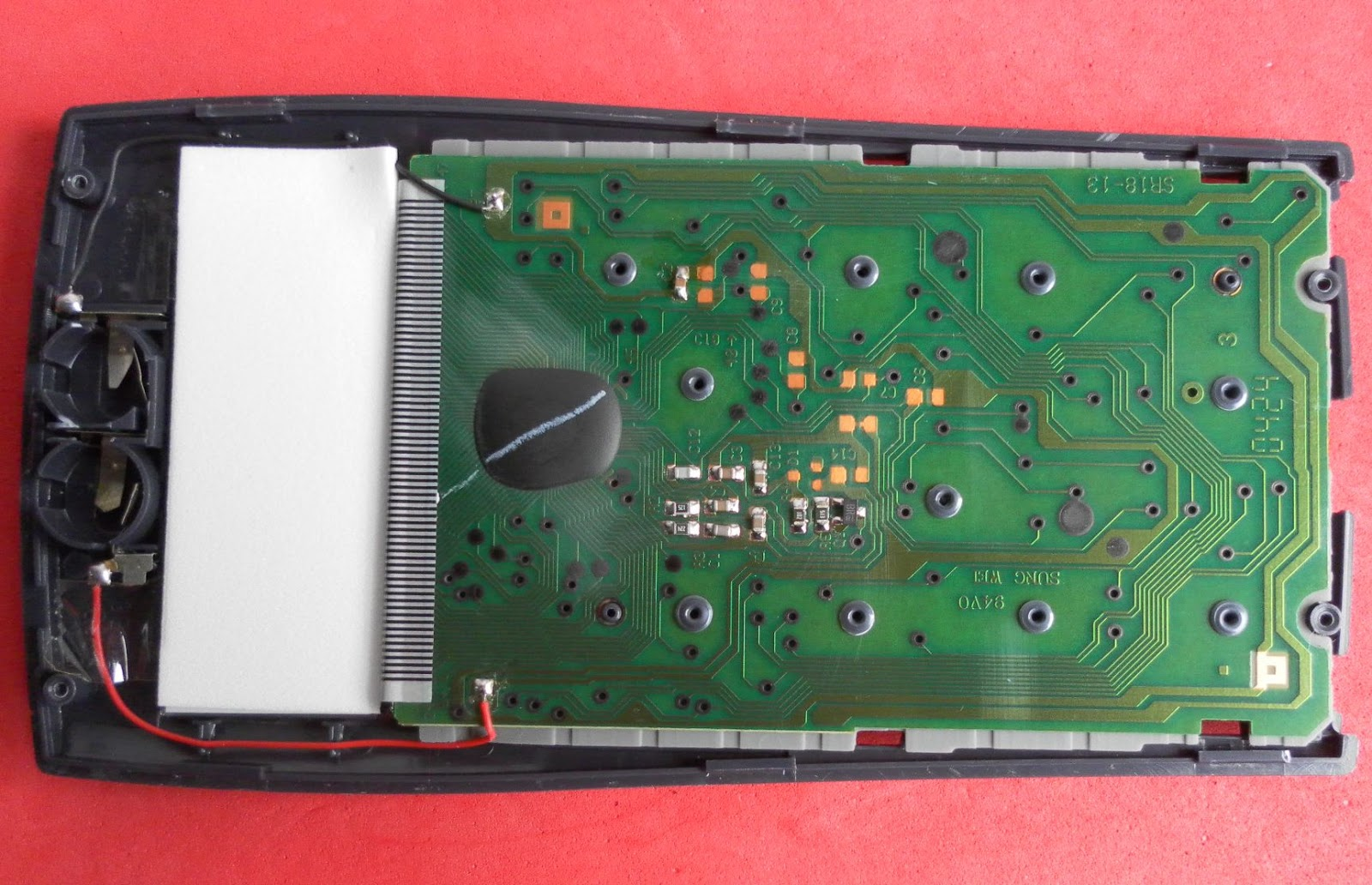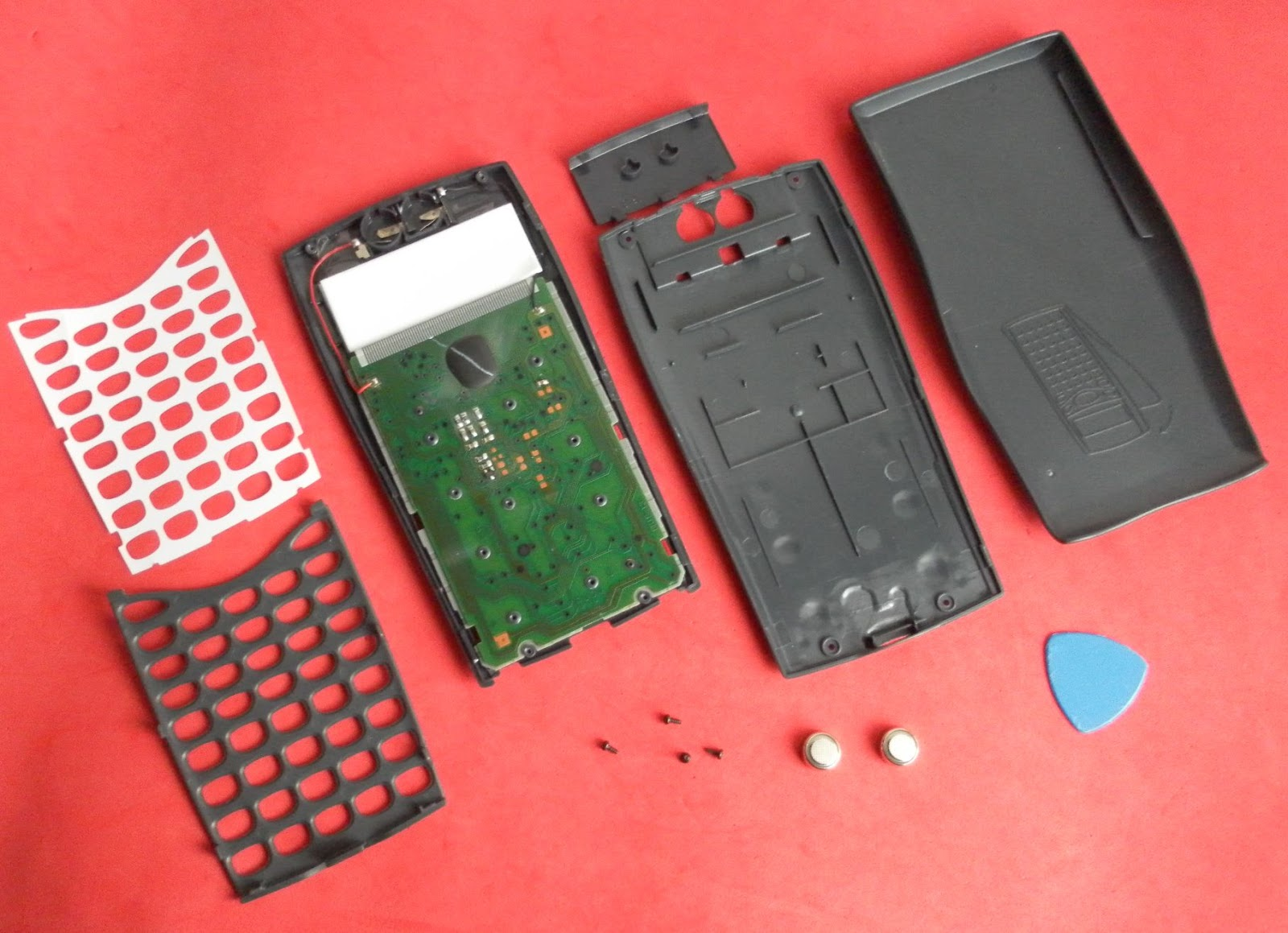HP-30S Scientific Calculator
01-26-2017, 11:47 PM
Post: #1jebemSenior Member Posts: 1,326 Joined: Feb 2014
HP-30S Scientific Calculator
I already had a few HP-35S along with HP-33S but no HP-30S.
So I bought this used 30S for a few Euros just to complete the series.

It was hard to find the proper HP documentation but after so much searching eventually I managed to get the Learning Modules to complement the succinct user guide.
The HP site seems to ignore this model altogether, despite having all the documentation stored but not listed anywhere. And because HP web servers naturally don't allow you to browse the url directories, the only way to find something is to use Google to look for the correct title of each document.

Official HP information
Release date: 2000
HP codename: Astro

CPU: Sunplus SPLB22A 8-bit CMOS
Power 2 x LR 44 batteries
LCD Display: 2 lines x 10 characters, Contrast Adjustable

Entry-system: Algebraic
History stack records all the entries up to a maximum 320 characters.

10 Memory registers, constant memory.
Last Entry memory.
Constant expression [K] memory.
Running memory [M].
EQN variable to store and execute expressions.

Largest number: 9.999999999E99.
Smallest non-zero positive displayed number: 1E-99
Internal smallest positive number: 10E–100.
Internal precision: 24 digits
Internal π constant displays an approximation to 21 decimal digits: 3.141592653589793238462.

HP Documentation
Besides the small succinct "user guide", HP have released a nice set of Learning Modules.

HP 30S Introduction to the Learning Modules
Being determined to keep up this tradition, HP provides these learning modules to help readers learn about the HP 30S, or to gain experience in its use.
Readers who do not have an HP 30S but wish to learn about it can benefit by studying these aids too.

HP 30S Basic Arithmetic
Computations are done using the HOME mode, although the STAT, L SOLV and Q SOLV can also be used.
The largest number the HP 30S can represent is 9.999999999E99. The smallest non-zero positive number this calculator can display is 1E-99. Internally, the smallest number is 10E–100.
Results greater than 10^10 or less than 10^–9 are displayed in scientific notation.
Implicit multiplication.

HP 30S Clearing, Editing and Correcting
Calculation history, command line editor.

HP 30S Operating Modes and Display Format
Modes: 0) HOME, 1) STAT, 2) L SOLV and 3) Q SOLV
Display formats: Floating Point, Scientific, Engineering. Fixed with up to 9 decimal places.

HP 30S Using the Built-in Physical Constants
The CONST menu provides twelve physics constants expressed in SI units.

HP 30S Solving Problems Involving Unit Conversions
SI metric units and Imperial units. The CONV menu provides nine pages of functions for converting to and from metric units.

HP 30S Solving Problems Involving Fractions

Decimal-to-fraction conversion.

HP 30S Using Memories to Solve Problems

The history stack and the last answer functions.
The history stack is a record of all the entries made by the user, up to a maximum 320 characters.
The VRCL menu lists 11 variables: A, B, C, D, x1, x2, X, Y, y1, y2 and EQN.
Running memory M+, M-.
Constant expression (K).

HP 30S Powers and Roots
0^0 is an error condition (Domain Error) because is mathematically an indeterminate form, much like 0/0 or log 0.

HP 30S Solving Problems Involving Percents

HP 30S Logarithmic Functions

HP 30S Converting Angles and Times
Convert between Radians, Degrees and Grads. Convert between decial degrees and DMS.

HP 30S Solving Trigonometry Problems

In Radians mode, Sine of π = 0.
Indeed, the sine of the irrational number π (which has an infinite number of significant digits) is zero, but π actually returns an approximation to twenty-one decimal digits: 3.141592653589793238462 (note 1).

Is the sine of that number smaller than 10E-99?
If so, the HP 30S would automatically substitute the number zero.
But, that’s not the case, the sine in question is approximately -5E10-19.

So, what’s happening? The HP 30S evaluates to 0 the sine of any number x such that:
3.141592653589793237976281≤ x ≤ 3.141592653589793238945133.

That’s not exactly cheating, but a way of producing exact answers by implementing a very important property of π, which is that its sine is zero.
The question was: what is the sine of π?
Will you ever interested in the sine of 3.141592653589793238462?

Note 1: Numbers with more than twelve significant digits can be entered by splitting them: e.g. 3.14159265358 + 9.79323893E-12.

HP 30S Hyperbolic Functions

HP 30S Polar/Rectangular Coordinate Conversions

The HP 30S provides four functions for converting between polar and cartesian coordinates. They are in the R↔P menu.

HP 30S Working with Expressions

The HP 30S provides a way of evaluating an expression containing one or more variables for various values. An entire expression can be stored as in the EQN variable, which, when retrieved and executed, will prompt you for values of all the variables used in the expression.

HP 30S Solving Linear Systems
The L SOLV mode is an operating environment in which systems of two linear equations in two variables can be solved easily.

The Q SOLV mode is an operating environment in which the quadratic equation ax^2+bx+c=0 can be solved to find the real solutions (if any).
If the error message is NO REAL SOL, then the two complex solutions can be calculated by using adequate expressions that can be automated using the EQN variable.

HP 30S Solving Problems Involving Complex Numbers
The HP 30S has no specific functions for operating with complex numbers.
This calculator is powerful enough to carry out calculations with complex numbers easily by using the R↔P menu. Also one can use expressions in Q SOLV and L SOLV modes to automate the process of finding the complex roots of a quadratic polynomial.

HP 30S Base Conversions
In the HP 30S, there is no specific operating mode to operate with binary, hexadecimal or octal numbers. However it is possible to automate the convertion between base systems by storing a expression in the EQN variable.

HP 30S Statistics – Averages and Standard Deviations
Average, Sample and population standard deviations, 1-VAR and 2-VAR stats.

HP 30S Statistics – Linear Regression

On the HP 30S, linear regressions are calculated in 2-VAR STAT operating mode.

HP 30S Probability – Random Numbers
The HP 30S provides two commands to generate random numbers, namely RANDM and RANDMI. The former returns a random number between 0 and 1, and the latter takes two integers A and B and returns a random integer n such as A ≤ n ≤ B.

HP 30S Probability – Rearranging Items

The PRB menu has the factorial, permutations and combinations functions.

HP 30S Solving Compound Interest Problems
Even though the HP 30S is a scientific calculator, it can solve a wide variety of compound interest problems.What several experts said at the time on the internal architecture

It uses binary arithmetic instead of BCD to perform floating point calculations.
Traditional HP calculators used BCD, allowing for a almost direct conversion of the internal values to be presented as decimal numbers in the display.
By using internal binary arithmetic with 80 bit precision (up to 24 digits in decimal), this calculator uses binary to decimal conversion and a final rounding before presenting the result in the 10 digit display.

Self-test.
Press 2nd Reset, followed by holding 2nd +/- Del to get the menu: TEST:1)D2)K
Press 2 to test the keyboard. 48 columns, one for each key to test are displayed.

Note the "04" value at the right side of the self test menu. Could it be the firmware version?What several experts said at the time on firmware releases.
Apparently there are at least two different firmware releases. The initial series had a few calculation issues and behaviors that were later silently corrected and new behaviors introduced by Kinpo/HP.

Operation modes. Home, Stat, Linear Solver and Quadratic Solver.The usual forensics test arcsin(arccos(arctan(tan(cos(sin(9))))) returns exactly 9.
Subtracting 9 from the Answer the result again is exactly Zero.Brief check for sqrt(2) = 1.4142135623730950488What several experts said at the time on specific calculation behaviors.

This section was collected mainly from discussions posted by several MoHPC members along the years:
http://www.hpmuseum.org/cgi-sys/cgiwrap/...5973#85973
http://www.hpmuseum.org/cgi-sys/cgiwrap/...8282#88282
http://www.hpmuseum.org/cgi-sys/cgiwrap/...3934#83934
http://www.rskey.org/hp30s

Depending on the firmware release (based on the serial number batches), it can present one or another of these behaviors.

- In Radians mode, the Sin(π) = 0 exactly.
This is a documented behavior by HP.
"On a Saturn-processor calculator, take "sin 3.14159265358" in radians mode. You will get the numerically-correct result of 9.79323846264 x 10-12 - which not-coincidentally are the next 12 significant digits of pi, given that the input was not, in fact, exactly pi.
Now, try the same on the HP-30S. Start with "sin 3.1415926535". The displayed result is 8.979323846 x 10-11 -- correct to its 10-digit display.
Then try "sin 3.14159265358". The answer returned is exactly zero! What happened? Rounding, for the sake of reassurance -- "This answer must actually be zero, so let's return that result to gratify the user."

- On the initial production batches, the Square root is only accurate to 11-12 digits.
To fix, compute the average of square root(x) and x/square root(x).
However, some noticed that the later production batches returns a correct 24 digit square root.

- Transcendental functions is only accurate to 14-18 digits.

The HP 30s having later serial numbers like CN0143, CN0303 or even later CNA 63400675 (circa 2008), calculates the cos(1.57079632) to 6.794896619231321e-9.
To get this result: COS(1.57079632)*1E5*1E5*1E7-679489661 to get the result of .92313212.
This is the correct answer to 16 significant figures making these one of the most accurate calculators to bear the HP logo.
Someone noticed that SIN(3.14159265358) returns exactly zero on the later serial numbers.

However, some early calculators batches with serial numbers like CN0019 will get a different result:
COS(1.57079632)*1E17-679489661 ENTER will get .923035657 which is the result with the leading digits 679489661 missing. So the actual result of COS(1.57079632) is: 6.79489661923035657E-9, only 12 accurate digits.
It appears that this could be a result of a rounding error by multipyling by 10e17 instead of 1E5*1E5*1E7, but that is not the case.
Apparently there are different firmware releases for different production batches.
Someone noticed that on the old serial numbers, SIN(3.14159265358) returns 9.793238461E-12

The HP30S can only accept 13 digits as keyboard input, so to perform calculations on 24 digit inputs, one must do arithmetic in the input string. On the newer serial numbers, if we type:
sin(3.1415926535+7391741495627E-23)*1E17-1587582 enter = .3506383
which is more or less consistent with internal 80 bit (24 decimal digit) arithmetic.
But, if we type:
sin(3.1415926535+7391741495628E-23) enter, we get exactly zero.
So, if you get close enough to PI on input, they return a result as if the input was exactly PI.

- Basic arithmetic operations uses 24 digits of internal precision.
This seems to be true, but there are a few quirks.

Key in 1 [a b/c] 110 to put in 1/110 as a fraction via the [a b/c] key. The result is: 0/1. Or calculate 1/10-1/11 in fractional form, the result is 0/1.
But the internal value is correct (0.009090909..). Only the displayed rational value is wrong. Later production batches changed this behavior and under these conditions the machine only displays the irrational decimal value.

Try 2+.2+.2+.2+.2+.2-3 = 0
A correct answer considering this is a binary arithmetic calculator.

Now try 2+1e-9-2 = 0
A wrong answer for a 24 digit binary arithmetic machine able to display up to 10 digits.
So the machine works internally to 24 digits and appears to suppress to 10 (and sometimes to just 9).
The HP-9G do not have this issue.

- Forensic test arcsin(arccos(arctan(tan(cos(sin(9))))) result is exactly 9.
It seems that whenever the result of a calculation is an integer followed by .9999999995+, that is, the digits after the decimal point are nine 9's and a 5 plus just a few more non-zero digits, the HP30S just rounds up to the next integer.
It appears that whenever the digits after the decimal point are greater than .9999999995 or less than .0000000005, they get dropped.

Part of the reason that the HP-30S returns the exact answer of 9 for the forensics test is that the HP-30S takes the liberty of rounding results to integers that are in very close proximity, which provides answers that are reassuring to novice users.

Dismantling.To open the machine we just need to undo the four screws in the back cover, and then use a pry tool on the sides to unlatch the back cover.The PCB has got some silk screen text:
"SUNG WEI" and "SR18-13".

What several experts said at the time
This calculator was manufactured by Kinpo. It is a modified SR18.Jose Mesquita

01-27-2017, 06:31 AM
Post: #2
 Gerald HSenior Member Posts: 1,442 Joined: May 2014
RE: HP-30S Scientific Calculator
On my 30S, SrNr 0230,

"Key in 1 [a b/c] 110 to put in 1/110 as a fraction via the [a b/c] key. The result is: 0/1. Or calculate 1/10-1/11 in fractional form, the result is 0/1.
But the internal value is correct (0.009090909..). Only the displayed rational value is wrong. Later production batches changed this behavior and under these conditions the machine only displays the irrational decimal value."

produces correct fraction.

"Now try 2+1e-9-2 = 0"

shows 0.000000001, ie correct.
01-27-2017, 07:49 PM
Post: #3
 rprosperiSenior Member Posts: 4,107 Joined: Dec 2013
RE: HP-30S Scientific Calculator
(01-26-2017 11:47 PM)jebem Wrote:  I already had a few HP-35S along with HP-33S but no HP-30S.
So I bought this used 30S for a few Euros just to complete the series.

Excellent and useful post Jose, everything I could want to know on this machine, all in one place. <bookmarked!>

Thanks for the work to collect and share all this 30s info. Too bad it's such a flimsy feeling machine; I've never used it enough to find it's bugs or quirks. It's also surprising HP invested so much effort on the documentation, but I guess it did form the base for the follow-on 33S and 35S.

--Bob Prosperi
01-28-2017, 12:58 AM
Post: #4jebemSenior Member Posts: 1,326 Joined: Feb 2014
RE: HP-30S Scientific Calculator
(01-27-2017 06:31 AM)Gerald H Wrote:  On my 30S, SrNr 0230,

"Key in 1 [a b/c] 110 ....."
produces correct fraction.

"Now try 2+1e-9-2 = 0"
shows 0.000000001, ie correct.

If the number "04" I mentioned above is related to the firmware release, then HP has issued at least 3 firmware updates during the machine life cycle, explaining the different results for the same tests.

(01-27-2017 07:49 PM)rprosperi Wrote:  Too bad it's such a flimsy feeling machine; I've never used it enough to find it's bugs or quirks. It's also surprising HP invested so much effort on the documentation, but I guess it did form the base for the follow-on 33S and 35S.

Thanks for your encouragement to keep doing this.
I find the HP Learning Modules very useful to learn on how to use the calculator.
Being machines primarily oriented to the schools, it makes sense to invest on documentation showing good math examples that the pupils/students can use.

Meanwhile I have found out that the HP-10S also have a set of Learning Modules.
Again, HP web servers do not have any links to access this information anymore, it is a tedious and time consuming task retrieving the files.

Jose Mesquita

05-28-2020, 06:08 PM (This post was last modified: 05-28-2020 06:26 PM by watchmaker.)
Post: #5
 watchmakerJunior Member Posts: 7 Joined: Aug 2018
RE: HP-30S Scientific Calculator
I've got an HP-30S with Version 03! S/N CN0024 (very early?)

1 ab/c 110 -> 0/1 :-D
2 + 1e-9 - 2 -> 1e-09
sin 3.14159265358 -> 9.793238461e-12
sin(3.1415926535+7391741495627E-23)*1E17-1587582 -> 3.504446358e-01
sin(3.1415926535+7391741495628E-23)*1E17-1587582 -> 3.504438966e-01 :-O

Quote:Part of the reason that the HP-30S returns the exact answer of 9 for the forensics test is that the HP-30S takes the liberty of rounding results to integers that are in very close proximity, which provides answers that are reassuring to novice users.
Very likely! Check Citizen SRP-280 and SRP-285II (other models from the same Kinpo series). The 280 gives approx. 5.2e-11 in the forensics test, the 285II (and all later Citizen models) gives 0.
Note, SRP-285 (without II!) is 14-digit decimal, not binary. TI models are also decimal.

Quote:Key in 1 [a b/c] 110 to put in 1/110 as a fraction via the [a b/c] key. The result is: 0/1. Or calculate 1/10-1/11 in fractional form, the result is 0/1
Citizen models (280, 285, 285II, 300) and TI-34II (also a similar Kinpo model!) do this fine. Maybe it's a specific firmware bug in HP-30 v. 03.
05-28-2020, 06:25 PM
Post: #6
 watchmakerJunior Member Posts: 7 Joined: Aug 2018
RE: HP-30S Scientific Calculator
Quote:On the initial production batches, the Square root is only accurate to 11-12 digits.
CONFIRMED! SQRT(2) is accurate only to 12 digits! Citizen SR-19 binary models (SRP-280, SRP-300 etc.) give at least 20 digits.
 « Next Oldest | Next Newest »

User(s) browsing this thread: 1 Guest(s)